# DrydenContinuousTurbulence

Demonstrates how to model wind turbulence for aircraft with the BandLimitedWhiteNoise block (a simple model of vertical Dryden gust speed at low altitudes < 1000 ft)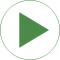# Information

This information is part of the Modelica Standard Library maintained by the Modelica Association.

This example shows how to use the BandLimitedWhiteNoise to feed a Dryden continuous turbulence model. This model is used to describe turbulent wind at low altitudes that varies randomly in space (see also wikipedia).

#### Turbulence model for vertical gust speed at low altitudes

The turbulence model of the Dryden form is defined by the power spectral density of the vertical turbulent velocity: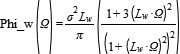The spectrum is parametrized with the following parameters:

• Lw is the turbulence scale.
In low altitudes, it is equal to the flight altitude.
• sigma is the turbulence intensity.
In low altitudes, it is equal to 1/10 of the wind speed at 20 ft altitude, which is 30 kts for medium turbulence.
• Omega is the spatial frequency.
The turbulence model is thus defined in space and the aircraft experiences turbulence as it flies through the defined wind field.
• Omega = s/V will be used to transform the spatial definition into a temporal definition, which can be realized as a state space system.
• V is the airspeed of the aircraft.
It is approximately 150 kts during the approach (i.e. at low altitudes).

Using spectral factorization and a fixed airspeed V of the aircraft, a concrete forming filter for the vertical turbulence can be found as,

for which V * (H_w(i Omega/V) * H_w(-i Omega/V) = Phi_w(Omega).

#### The input to the filter

The input to the filter is white noise with a normal distribution, zero mean, and a power spectral density of 1. That means, for a sampling time of 1s, it is parameterized with mean=0 and variance=1. However, in order to account for the change of noise power due to sampling, the noise must be scaled with sqrt(samplePeriod). This is done automatically in the BandLimitedWhiteNoise block.

#### Example output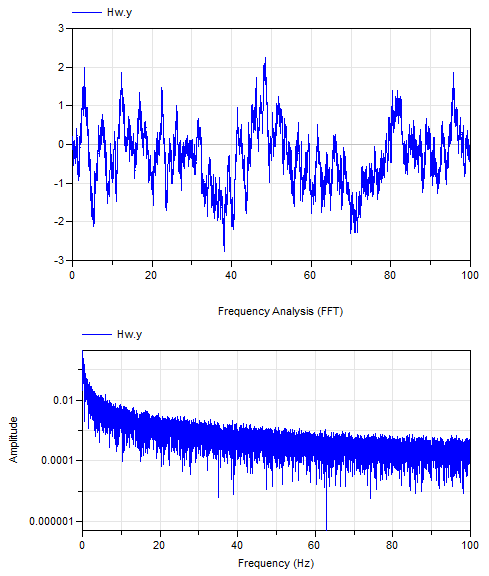#### Reference

1. Dryden Wind Turbulence model in US military standard MIL-F-8785.

# Parameters (3)

V Value: 140 * 0.5144 Type: Velocity (m/s) Description: Airspeed of aircraft (typically 140kts during approach) Value: 0.1 * 30 * 0.5144 Type: Velocity (m/s) Description: Turbulence intensity (=0.1 * wind at 20 ft, typically 30 kt) Value: 600 * 0.3048 Type: Length (m) Description: Scale length (= flight altitude)

# Components (4)

Hw whiteNoise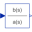Type: TransferFunction Description: Transfer function of vertical turbulence speed according to MIL-F-8785C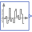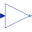Type: Gain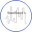Type: GlobalSeed